7 min read# Corrosion current measurement for an iron electrode in an acid solution (Tafel plot LPR) Corrosion- Application Note 10

Latest updated: May 2, 2023

Abstract

The characterization of a corrosion system depends on the determination of cathodic and anodic Tafel parameters (βc, βa) and the corrosion rate. The measurement of these parameters are mainly determined using the Linear Polarization (LPT) and Tafel Plot (TP) techniques available in EC-Lab software.

In this note, iron in acidic media was characterized as a tafelian system. The corrosion parameters were determined using Stern (TP) and stern and Geary (LPT) methods. The corrosion rate value was obtained with Tafel Fit was compared to that obtained with Rp Fit.

## Introduction

The corrosion current is a typical corrosion value which can be related, for example, to the corrosion rate. The information obtained from both values is necessary when studying the corrosion state of a given system. The aim of this tutorial is to demonstrate to the user how to determine the corrosion current using simple graphic tools on EC-Lab® linked to the Tafel equation: Tafel fit and Rp fit.

## Experimental Conditions

• Working electrode: RDE (Rotating Disk Electrode) of iron, working area: 0.0314 cm2, electrode rotation speed: Ω = 800 rpm (rotations per minute).
• Counter electrode: Platinum wire
• Reference electrode: Saturated Calomel Electrode (SCE)
• Solution: HCl (0.1 M)

## Protocol Description

The current was measured through a LSV (Linear Sweep Voltammetry) response with a low scan rate (10 mV/s). The potential was scanned from -0.6 to 0 V/SCE.

The protocol used under EC-Lab was Linear Polarization (Fig. 1). The parameters settings were the following:

In the “Parameters Settings” tab,

• 1st block: default settings
• 2nd block:
• Scan EWE with dE/dt = 50 mV/s from EI = -0.6 V <None> to EL = 0 V vs. <None>
• Record <I> over the last 100 % of the step duration, average N = 50 voltage steps
• with I Range = Auto and Bandwidth = 5 – medium

Note: In the “Advanced Settings” tab, EWE max and EWE min were respectively set to +1 and -1 V. This increases the potential control resolution (span) by diminishing the minimum potential step height from 300 to 50 μV.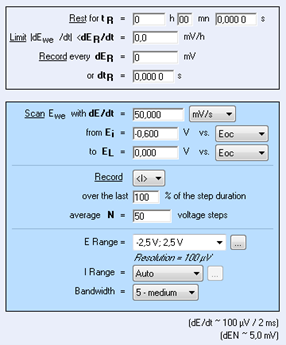Figure 1: LP “Parameters Settings” window.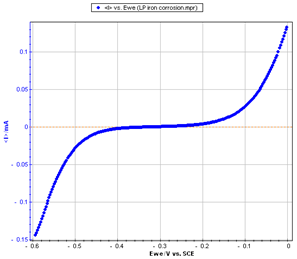Figure 2: Steady-state curve I vs. EWE

Note: It is possible to load the different LP_iron_corrosion files with EC-Lab software in the following folder:
C:\Users\…\Documents\EC-Lab\Data\Samples\Corrosion

## Stern Method (Tafel Fit)

The Stern relation can be written as followed:

$$I = I_{corr}\left(\mathrm{exp}\left(\mathrm{ln}10\frac{E-E_{corr}}{\beta_a}\right)\mathrm{-exp}\left(\mathrm{ln}10\frac{E-E_{corr}}{\beta_c}\right)\right) \tag{1}$$

From the graph displaying log |I| vs. EWE, it is possible to determine the values of Icorr, Ecorr, βa and βc by a simple analysis.
The Tafel fit, which is a Graph Analysis tool from EC-Lab®, can determine automatically these values (Fig. 3).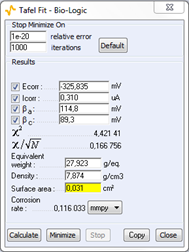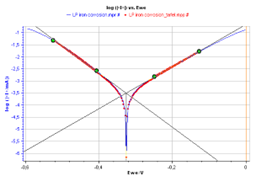Figure 3: Tafel Fit Analysis.

Note: The corrosion rate can be determined if the user enters the values of the equivalent weight (atomic weight divided by the number of electrons involved in the reaction), the material density and the active surface area.

## Stern and Geary Method (Rp Fit)

The expression of the polarization resistance can be defined as:

$$R_P = \frac{1}{dI/dE} \tag{2}$$

Which gives, at $E= E_\mathrm{corr}$, using Eq. 1:

$$R_{p,E_{corr}}=\frac{\beta_a \beta_c}{I_{corr}(\beta_a + \beta_c)\mathrm{ln}10} \tag{3}$$

and knowing the values of Rp,Ecorr, βa and βc, we can figure out the value of Icorr with the following relationship:

$$I_{corr}=\frac{\beta_a \beta_c}{R_{p,E_{corr}}(\beta_a + \beta_c)\mathrm{ln}10} \tag{4}$$

The value of Rp,Ecorr can be simply determined by displaying the graph giving EWE vs. I around the corrosion potential and calculating the slope of the curve.

The Rp fit, which is a graph analysis tool from EC-Lab (cf. Quickstart – Analysis Graph Tools), can automatically calculate the value of Rp. It is also possible to determine the corrosion current Icorr if the values of the Tafel coefficients βa and βc are known. The user will just have to type these values in the Rp fit analysis window before the fit (Fig. 4).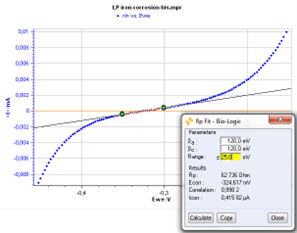Figure 4: Rp fit analysis.

## Conclusion

The values of the Tafel coefficients βa and βc found with the Tafel fit were used to find the corrosion current with the Rp fit and the results found with both methods are very close:

• for Tafel fit, Icorr = 0.310 μA
• for Rp fit, Icorr = 0.416 μA

These graphic tools seem therefore quite efficient. The Tafel fit can also be used to determine the corrosion rate. The value found for this experiment is:

Corrosion rate = 0.116 mmpy.

## References

1. D. Landolt, in : Traité des Matériaux, 12, Presses Polytechniques et Universitaires Romandes (Ed.), (2003).
2. J.P. Diard, B. Le Gorrec and C. Montella, in: Cinétique électrochimique, Hermann, (1996) 204.

Revised in 07/2018

Work smarter. Not harder.

Tech-tips, theory, latest functionality, new products & more.

No thanks!